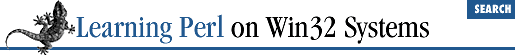home | O'Reilly's CD bookshelfs | FreeBSD | Linux | Cisco | Cisco Exam

### A.14 Chapter 15, Other Data Transformation

1. Here's one way to do it:

```while (

<>) {
chomp;
\$slash =

rindex(\$_,"/");
if (\$slash > -1) {

substr(\$_,0,\$slash);
\$tail = substr(\$_,\$slash+1);
} else {
}
}```

Each line read by the diamond operator is first ``` chomp``` ed (tossing the newline). Next we look for the rightmost slash in the line, using ``` rindex()``` . The next two lines break the string apart using ``` substr()``` . If no slash exists, the result of the ``` rindex``` is ``` -1``` , so we hack around that. The final line within the loop prints the results.

2. Here's one way to do it:

```chomp(@nums = <STDIN>); # note special use of chomp
@nums =

sort { \$a <=> \$b } @nums;
foreach (@nums) {
printf "%30g\n", \$_;
}```

The first line grabs all of the numbers into the ``` @nums``` array. The second line sorts the array numerically, using an inline definition for a sorting order. The ``` foreach``` loop prints the results.

3. Here's one way to do it:

```

while (<>) {

substr(\$_,0,1) =~ tr/a-z/A-Z/;
substr(\$_,1) =~ tr/A-Z/a-z/;
print;
}```

For each line read by the diamond operator, we use two ``` tr``` operators, each on a different portion of the string. The first ``` tr``` operator uppercases the first character of the line, and the second ``` tr``` operator lowercases the remainder. The result is printed.

Another way to do this, using only double-quoted string operators, is:

```while (<>) {
print "\u\L\$_";
}```

Give yourself an extra five points if you thought of that method instead.A.13 Chapter 14, Process ManagementA.15 Chapter 16, System Information